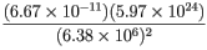# Problem: A number written in scientific notation has the form a x 10^{k}, where 1 &lt; a &lt; 10 and k is an integer, that is, k is one of ... -3, -2 , -1, 0, 1, 2, 3.... Consider the expression  Determine the values of a and k when the value of this expression is written in scientific notation. Enter a and k, separated by commas.

###### FREE Expert Solution

(6.67 × 10-11)(5.97 × 1024)/(6.38 × 106)2 = 9.7827###### Problem Details

A number written in scientific notation has the form a x 10^{k}, where 1 < a < 10 and k is an integer, that is, k is one of ... -3, -2 , -1, 0, 1, 2, 3....

Consider the expressionDetermine the values of a and k when the value of this expression is written in scientific notation. Enter a and k, separated by commas.